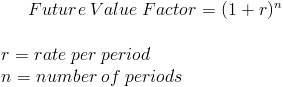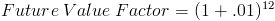# Future Value FactorThe formula for the future value factor is used to calculate the future value of an amount per dollar of its present value. The future value factor is generally found on a table which is used to simplify calculations for amounts greater than one dollar (see example below).

The future value factor formula is based on the concept of time value of money. The concept of time value of money is that an amount today is worth more than if that same nominal amount is received at a future date. Any amount received today can be invested and receive earnings, as opposed to waiting to receive the same amount with no earnings. An amount of \$105 to be received a year from now may be okay if the individual wants \$100 today, assuming that the individual can earn 5% otherwise in one year.

## Rate Per Period/Number of Periods

It is important when using the formula for the future value factor to match the rate per period with the number of periods. The number of periods should also match how often an investment is compounded. For example, assume that the nominal interest rate is 12% per year compounded monthly. Since this account is compounded monthly, then 1% per month would be used in the formula to calculate the future value factor.

## Example of Future Value Factor Formula

Using the prior example of 12% compounded monthly, the future value factor formula for one year would showwhere 1%, or .01, is the rate per period and 12 is the number of periods. By solving this equation, the future value factor for 12 periods at 1% per period would be 1.1268.

As previously stated, the future value factor is generally found on a table that is used for quick calculations for amounts greater than one dollar. With this example, assume that an individual is attempting to calculate the value after one year for the amount of \$500 today based on a 12% nominal annual rate compounded monthly. By looking at the future value factor table, the individual would find 1.1268. Since this factor is based on \$1, the factor can then be multiplied by the \$500 to find a future value of \$563.40.

New to Finance?